# Group theory

(Redirected from Free group)

In mathematics, groups often arise as structures representing the set of possible symmetries of some object. We have been intentionally vague about the meaning of the terms symmetry and object. The terms may have an obvious geometric sense. For example, the set of orientation-preserving continuous transformations of the Euclidean plane$\mathbb{R}^2$ that take lines to lines and preserve angles can be decomposed into transformations of two types: rotations which rotate the whole plane around the origin by an angle of θ, and translations, which shift the origin to a new point and move the whole plane along with it without any "twisting". In coordinates, we can write an arbitrary rotation as$(x, y) \mapsto (x\cos\theta - y\sin\theta, x\sin\theta + y\cos\theta)$,

and translations as$(x, y) \mapsto (x + x_0, y + y_0)$.

Of course, rotations and translations can be combined, and the full group of symmetries is called the 2-dimensional affine group. Felix Klein pioneered this approach to geometry, calling it the Erlangen Program. It would be subsequently taken up and generalized by Élie Cartan and remains an important approach to differential geometry.

But it would be misleading to focus entirely on geometric examples. In fact, the very word group was introduced by Évariste Galois in the context of algebraic equations, as part of what is now called Galois theory. Consider the polynomial x2 + 1, it is irreducible over the real numbers, meaning that we cannot find two real numbers a and b, such that

x2 + 1 = (xa)(xb).

However, over the complex numbers we can write

x2 + 1 = (x + i)(xi).

Now, complex conjugation, that is, the mapping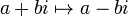$a + bi \mapsto a - bi$, can be thought of as a "symmetry" of the complex numbers. Now, what happens if we take the complex conjugate of the constant values in (x + i)(xi)? We get, (xi)(x + i); that is, the order of the factors is reversed, but when we multiply it out, the product is still x2 + 1. It turns out that complex conjugation and doing nothing are the two "symmetries" of the complex numbers having the property that a) the ordinary algebraic operations of addition, multiplication, taking reciprocals and multiplying by a real number and b) the polynomial x2 + 1 are left unchanged. This two element group (written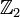$\mathbb{Z}_2$) is called the Galois group of the extension$\mathbb{C}/\mathbb{R}$ or, more simply, of the polynomial x2 + 1. This is but one example of how groups can arise in mathematics where we are not directly concerned with symmetries of geometric objects.

##  Definitions of groups and subgroups

###  Groups

A group is a set G and a binary operation$\cdot$ that has the following properties:

• The group has an identity element: There is an element e, such that$x \cdot e = x$ and$e \cdot x = x$ for all x in the group.
• Every element has an inverse: For each element x in the group, there is another element y, such that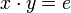$x \cdot y = e$ and$y \cdot x = e$. (e is the identity element)
• The operation is associative: For all elements x, y and z we have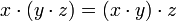$x \cdot (y \cdot z) = (x \cdot y ) \cdot z$.

###  Subgroups and normal subgroups

A subgroup is a subset of a group that is itself a group with respect to the "inherited" operations. Not every subset of a group is a subgroup (for example, a subset that does not contain the identity element e cannot be a group). A normal subgroup is a very important kind of subgroup and is defined by a few different equivalent definitions. The role of normal subgroups will be shown in the next few sections.

##  Special kinds of groups

An abelian group is a group with an operation that is commutative. That is,$x \cdot y = y \cdot x$ for every element x and y in the group. Abelian groups are often easier to analyze than non-abelian groups. For example, all subgroups of an abelian group are normal. As a matter of style, the operator on an abelian group is often called "addition" and the identity element called 0. Conversely, non-abelian groups often (but not always) use the multiplication operator$\cdot$ and call the identity element 1. Again, the notation used is a matter of context and preference.

A cyclic group is a group that is generated by a single element. The cyclic group G generated by the element g is the set of all the integral powers of the element g and its inverse. Every cyclic group is abelian, but the converse is not true (see the examples).

A solvable group, or a soluble group, is a group that has a normal series whose quotient groups are all abelian. A simple group is a group that has no normal subgroups, except for the trivial subgroup and the group itself. One interesting simple group is the alternating subgroup A5, which has 60 elements. Simple groups, except for the cyclic groups of prime order, cannot be solvable, and so A5 and the symmetric group S5 are not solvable. This is one of the first important results to arise from group theory. The fact that S5 is not solvable gives a proof that there is no closed form solution to solve a 5th-degree polynomial (recall that the formula for the solution of a quadratic equation gives the roots for any 2nd-degree polynomial; this result states that there is no such formula for a 5th-degree polynomial, or any other general polynomial with degree larger than 4). Most finite simple groups fall in series. It was eventually proved that there are exactly 26 others, called sporadic. The proof of this was spread through many papers totalling about 15,000 pages.

A free group is a group in which there is a subset such that every element of the group can be written uniquely as the product, or string, of elements of the subset (and their inverse elements). Every group is isomorphic to a quotient group of some free group, so understanding the properties of free groups helps us understand the structure of all groups. Free groups are also used to find the presentation of a group, a useful tool used to completely characterize the structure of a group.

##  Examples of groups

###  Abelian examples

The integers together with addition is a cyclic group. Consider the required properties:

• Addition is a well-defined binary operator. Whenever a and b are integers, a + b is also an integer, and so the operation is closed on the group.
• The element 0 is the additive identity for the group. It is easy to see that a + 0 = a and 0 + a = a.
• Every element has an inverse. For example, the inverse of 2 is -2, because 2 + ( − 2) = 0.
• Addition is associative. (a + b) + c = a + (b + c)
• The group is abelian. a + b = b + a
• The group is cyclic with 1 as its generator. For example, 2=1+1, 3=1+1+1, 4=1+1+1+1, and so on. Further, -3=(-1)+(-1)+(-1), the third multiple of the inverse of 1.

By similar reasoning, the real numbers with addition are an abelian group, but not cyclic. There is no single element such that every real number is some integral multiple of that element (if you suppose that there is such an element, say a, then a/2 is not an integral multiple of a).

The integers with multiplication, however, is not a group. Multiplication is well-defined and associative, and 1 is an identity element. However, not all elements have inverses. For example, the inverse of 2 should be 1/2 (since$2\cdot\frac{1}{2}=1$), but 1/2 is not an integer.

However, the real numbers with multiplication are still not a group. It is almost a group, since all the nonzero real numbers have an inverse (the inverse of a is 1/a). But, zero has no inverse. This single failure means that the real numbers with multiplication are not a group.

###  Nonabelian examples

One of the first examples of non-commutative multiplication arises in linear algebra. Matrix multiplication is not commutative (the product AB is not the same as BA).

The set of all n-by-n invertible real matrices together with matrix multiplication is a group. This group is called the general linear group of degree n, and is written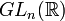$GL_n(\Bbb{R})$.

As a finite example, the symmetric groups of degree greater than 2 are all nonabelian.

##  Operations involving groups

###  Morphisms

A group homomorphism is a map from one group to another group that preserves the multiplicative structure of the groups; written formally, the map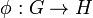$\phi:G \rarr H$ obeys the rule$\phi(a \cdot b) = \phi(a) \cdot \phi(b)$. The kernel of a homomorphism is the set of all elements that map to the identity element; this set is a normal subgroup, and every normal subgroup is the kernel of some homomorphism.

An embedding is an injective homomorphism (or, equivalently, one whose kernel consists only of the identity element). We say that the two groups are isomorphic if there is a surjective (thus bijective) embedding of one into the other (then the embedding is called an isomorphism). Isomorphic groups have identical structure and are often thought of as just being relabelings of one another. Isomorphisms are important because they allow us to think of the same group in different ways. For example, take a certain group and we could consider its isomorphic version in the symmetric groups (from Cayley's theorem), its isomorphic version as a quotient group of a free group, or as a group of matrices over some field (as a group representation).

An automorphism is an isomorphism of a group onto itself. The set of all automorphisms for a group G, often written Aut(G), is itself a group! One important subgroup of this automorphism group is the set of all inner automorphisms. An inner automorphism is a conjugation mapping (for an element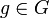$g \in G$, the mapping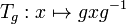$T_g: x \mapsto gxg^{-1}$ is an inner automorphism). The inner automorphism subgroup is isomorphic to the quotient group G / Z(G), where Z(G) is the center of the group. The inner automorphism subgroup is normal, indeed characteristic, inside Aut(G), and the quotient group Out(G) = Aut(G) / Inn(G) is called the outer automorphism group. Finding non-inner automorphisms for a group is, in general, difficult. Because abelian groups have a trivial inner automorphism subgroup, finding automorphisms for abelian groups is, strangely enough, harder than for nonabelian groups.

###  Group actions

A group action is a homomorphism of a group to the permutation group of a set. Group actions are incredibly useful. For example, the Sylow theorems are easily proved by considering a group acting on the set of its maximal p-subgroups. Group actions also give rise to the so-called orbit-stabilizer theorem, a very powerful counting theorem. As an application of this, it is easy to show that every finite p-group must have a non-trivial center by considering how a p-group acts on itself via conjugation.

##  Constructing groups from other groups

There are a few tools in group theory that give us ways to construct new groups from other groups.

A quotient group G / N is the set (and group) of cosets of a normal subgroup N of a group G. There is a canonical homomorphism from G onto the quotient group (for which N is the kernel), and so multiplication in the quotient group is related to multiplication in the original group. However, it is important to note that the quotient group is an entirely new group. There is no reason to expect (in general) that there is a subgroup of G that is isomorphic to the quotient group.

The direct product is another way to construct new groups. In the case of two groups G and H, the direct product$G \times H$ is the set of all tuples (g,h) where each component comes from G and H, respectively. Multiplication is done component-wise. Direct products result in groups that are "larger", in the sense that there is an isomorphic copy of both G and H in the direct product. When the groups are abelian, it is common to call the direct product as the direct sum.

The direct product of more than two groups is certainly possible, and the concept generalizes easily to any (finite or infinite) set of groups. However, in the case of infinite number of groups, there is an important distinction between direct sum and direct product. The tuples in an infinite direct sum are restricted to those that have a finite number of non-identity entries. Direct products have no such restriction.

The semidirect product is another generalization of the direct product in which the multiplication is not done independently in the components. The semidirect product$N \times_\phi H$ is the set of tuples (n,h) with components from two groups N and H, together with a mapping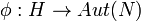$\phi:H \rarr Aut(N)$. This mapping distorts multiplication in the N coordinates, giving rise to a richer set of group constructions.

## Notes and references

1. A normal series is a tower of normal subgroups of a group, each one normal in the next (but not necessarily normal in the group itself):$\{e\}\triangleleft N_1\triangleleft \cdots \triangleleft N_n \triangleleft G$.
2. The quotient groups here are the Ni + 1 / Ni, using notation from the previous footnote.
3. It can be helpful to think of the subset as being "letters" in an alphabet and every element is a "word" over that alphabet. Every element of a free group has one and exactly one word that describes it (except that we do remove strings that are equivalent to the identity, so that a and abb − 1 are the same word.)
4. Notice also we say "normal SUBgroup" (because it is a subgroup) but never "quotient SUBgroup".Some content on this page may previously have appeared on Citizendium.